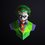# A pattern in $\tan x$

if we take a look at value of $\tan^{-1} 100$ , using a calculator we can say it is 89.427.
then if we go to $\tan^{-1} 1000$ , we get 89.9427
for $\tan^{-1} 10000$ it is 89.99427
$\tan^{-1} 100000$ is 89.999427.
So we have a pattern here using which we can predict the value of $\tan^{-1} 10^{n}$
for every zero after 100 , add a 9 after the decimal and let the 427 term remain same.
If anyone has an explanation please do share.Note by Mathoholic Oja
1 year, 4 months ago

This discussion board is a place to discuss our Daily Challenges and the math and science related to those challenges. Explanations are more than just a solution — they should explain the steps and thinking strategies that you used to obtain the solution. Comments should further the discussion of math and science.

When posting on Brilliant:

• Use the emojis to react to an explanation, whether you're congratulating a job well done , or just really confused .
• Ask specific questions about the challenge or the steps in somebody's explanation. Well-posed questions can add a lot to the discussion, but posting "I don't understand!" doesn't help anyone.
• Try to contribute something new to the discussion, whether it is an extension, generalization or other idea related to the challenge.

MarkdownAppears as
*italics* or _italics_ italics
**bold** or __bold__ bold
- bulleted- list
• bulleted
• list
1. numbered2. list
1. numbered
2. list
Note: you must add a full line of space before and after lists for them to show up correctly
paragraph 1paragraph 2

paragraph 1

paragraph 2

[example link](https://brilliant.org)example link
> This is a quote
This is a quote
    # I indented these lines
# 4 spaces, and now they show
# up as a code block.

print "hello world"
# I indented these lines
# 4 spaces, and now they show
# up as a code block.

print "hello world"
MathAppears as
Remember to wrap math in $$ ... $$ or $ ... $ to ensure proper formatting.
2 \times 3 $2 \times 3$
2^{34} $2^{34}$
a_{i-1} $a_{i-1}$
\frac{2}{3} $\frac{2}{3}$
\sqrt{2} $\sqrt{2}$
\sum_{i=1}^3 $\sum_{i=1}^3$
\sin \theta $\sin \theta$
\boxed{123} $\boxed{123}$

Sort by:

This is not hard to explain. First, the function you're trying to compute is actually $\frac{180}{\pi} \tan^{-1}(x),$ where the $\frac{180}{\pi}$ is just the factor that converts from radians to degrees.

First note that for $|x| < 1$ we have \begin{aligned} \frac{180}{\pi} \tan^{-1}(1/x) = 90 -\frac{180}{\pi}\tan^{-1}(x) &= 90 - \frac{180}{\pi} \left(x-\frac{x^3}3 + \frac{x^5}5 - \cdots \right) \\ &= 90 - \frac{180}{\pi} x + \frac{180}{\pi} \frac{x^3}3 - \frac{180}{\pi} \frac{x^5}5 + \cdots \end{aligned} and now we're plugging in $x = 1/10^n.$ Since $x^3, x^5, \ldots$ are tiny compared to $x,$ we can get a very good approximation by cutting off those terms. So we get \begin{aligned} \frac{180}{\pi} \tan^{-1}(10^n) &= 90 - \frac{180}{\pi} \frac1{10^n} + \frac{180}{\pi} \frac1{3 \cdot 10^{3n}} - \cdots \\ &\approx 90 - \frac{180}{\pi} \frac1{10^n} \\ &\approx 90 - \frac{57.3}{10^n} \end{aligned} and that's that. So: \begin{aligned} \frac{180}{\pi} \tan^{-1}(100) &\approx 90 - \frac{57.3}{100} = 89.427\\ \frac{180}{\pi} \tan^{-1}(1000) &\approx 90 - \frac{57.3}{1000} = 89.9427 \\ \frac{180}{\pi} \tan^{-1}(10000) &\approx 90 - \frac{57.3}{10000} = 89.99427 \\ \end{aligned} and so on.

- 1 year, 3 months ago

Hi! Just a couple of thoughts:

First, the function $\tan^{-1}(x)$ approaches $90^{\circ}$ as we input larger and larger numbers, so it makes sense that your values also get closer and closer to $90^{\circ}$ .

I actually tried a similar procedure with an initial input of $200$. I kept increasing this input by factors of $10$ and saw I similar pattern: just adding nines at the front of the decimal part. I suppose this would happen with most large numbers.

We can't exactly say that adding nines is all we have to do however, because the numbers you listed have been rounded. The remaining digits do change with each calculation.

I'm actually not quite sure though what causes this "add a nine" behaviour. Not sure if it is an actual mathematical property or just the result of using a calculator. Hopefully someone else knows!

- 1 year, 4 months ago

Hi David, Thanks for your info about the fact that it doesnt need to be factors of 10 to show this pattern and I know that I have taken an approximation but still it is mind boggling that we can begin predicting values of tan x where it starts getting unpredictable.

- 1 year, 4 months ago

Wow man What an observation, I cant even comprehend this

- 1 year, 4 months ago

Thanks

- 1 year, 4 months ago